# Davenport constant

(diff) ← Older revision | Latest revision (diff) | Newer revision → (diff)
Jump to: navigation, search

For a finite Abelian group, Davenport's constant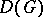ofis the smallest positive integersuch that for any sequence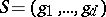of not necessarily distinct elements ofthere is a non-empty subsequence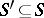with sum zero (i.e., there is somewith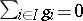).

It is reported in [a7] that in 1966 H. Davenport proposed the problem of findingin the following connection. Letbe an algebraic number field (cf. also Algebraic number; Field) with ring of integersand ideal class group. Thenis the maximal number of prime ideals (counted with multiplicity) which can divide an irreducible element of. This is the reason whyis a crucial invariant in the theory of non-unique factorizations [a2].

Let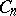denote the cyclic group withelements and suppose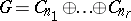with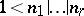and with rank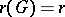. Then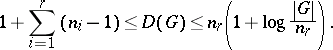In the left-hand inequality, equality holds for-groups and for groupswith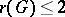(proved independently by J.E. Olson [a7] and D. Kruyswijk [a3]; cf. also-group). However, the left-hand inequality can be strict [a4], [a5]. For the right-hand inequality, cf. [a6]. It is still (1996) an open question whether the left-hand inequality can be strict for groups of rankor for groups of the form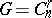.

The problem of determiningbelongs to the area of zero-sum sequences, a subfield of additive number theory, respectively additive group theory. For related problems cf. [a1] and the literature cited there.